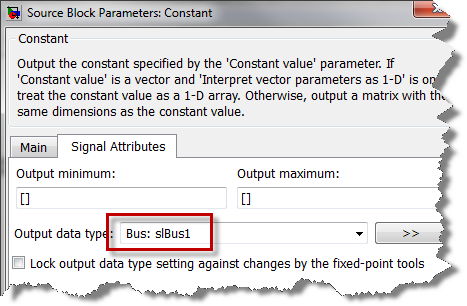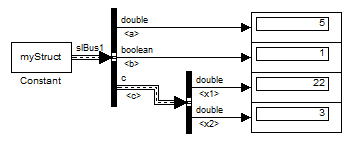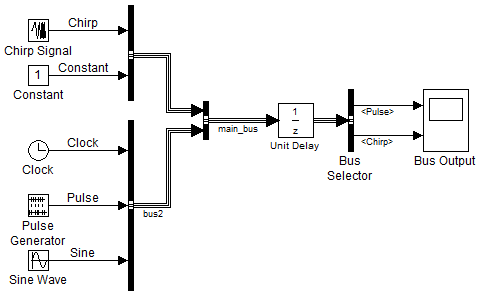# Initializing buses using a MATLAB Structure

Did you notice that since R2010a it is possible to initialize buses with a MATLAB structure? If you were not aware, here is how it works.

Starting from a Structure

Let's say I have a structure in the MATLAB workspace and I would like to make that a bus signal in Simulink. I can use Simulink.Bus.createObject to create a bus object:

 myStruct.a = 5; myStruct.b = true; myStruct.c.x1 = 22; myStruct.c.x2 = 3; busInfo = Simulink.Bus.createObject(myStruct); 

Then I can configure a Constant block to use this bus object as data type and to use myStruct as value.Starting from a model

Let's say I have the following model where a bus signal is going through a Unit Delay block. I want to specify different initial values for the elements of the bus.In that case I can use the handle to a port of the Unit Delay block as input to Simulink.Bus.createMATLABStruct. This will create a MATLAB structure with the same hierarchy as the bus, filled with zeros. I can then overwrite fields of this structure as needed and use it as the initial value of the Unit Delay.

 portHandle = get_param(gcb,'PortHandles'); initStruct = Simulink.Bus.createMATLABStruct(portHandle.Inport); initStruct.bus2.Pulse = 5; initStruct.signal1.Chirp = 3; 

Requirements to Use Bus Signal Initialization

To enable bus signal initialization, you need to set two Configuration Parameter diagnostics:

Even if you don't need to initialize buses, I always recommend using those setting. They help making your models easier to understand and improve the consistency of simulation results.

Now it's your turn

Look at Specifying Initial Conditions for Bus Signals for more details and let us know how you will use this feature by leaving a comment here.

|